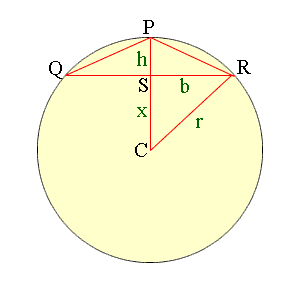Quandaries and Queries Hi, does anyone know the answer to this problem. We need a formula that we can use to figure this out for work. We aren't math wiz's or students. Basically we know 3 points in space of a triangle, we know the length of each side and the length of the line from apex to base line. Each point of the base line ends upon the circumference of a circle. IS three a way to work out the radius of that circle. Jynks Hi Jynks, I redrew the diagram you sent and labeled the vertices of the triangle as P, Q and R. C is the center of the circle, r is its radius, h is the height of the triangle, S is the midpoint of the base and b is one half the length of the base. I have used x for the unknown distance from C to S.The angle RSC is a right angle and hence Pythogoras' theorem for the triangle RSC gives x2 + b2 = r2 Also PC is a radius of the circle so r = x + h Thus x = r - h and substituting this into the first exprression and simplifying gives (r - h)2 + b2 = r2 r2 -2rh + h2 + b2 = r2 2rh = h2 + b2 r = (h2 + b2)/2h Cheers, Penny Go to Math Central